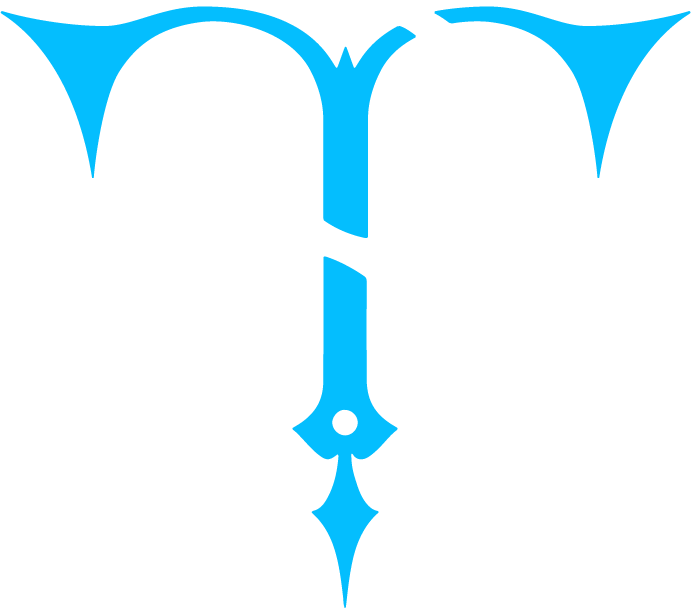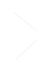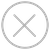TensorSpace.js
Getting StartBasic ConceptsTensorSpace ConverterModel PreprocessingModelsLayersMerge FunctionLayer Dimension
Definition
In machine learning, each layer all has a dimensional feature that is suffixed as 1d, 2d or 3d. It indicates the dimension of the layer output.
In TensorSpace, there are some special 2d layers which have a different output dimension as described above. For example, the "2d convolutional layer", extracts features by using different filters which generate feature maps. Let's say the input image size is 28x28, after passing through the 2d convolution layer, the output shape is [28, 28, 3] which has one extra dimension (channel) appended. The number of channels represents the number of filters.
Dimension of layer
In TensorSpace, the dimension of the intermediate layer is determined by the shape of the output data.
When we construct the network, we have to consider the detailed rules of computation. For example, after Input1d, we have to use pooling1d, not pooling2d.
One dimension layers
The output dimension is 1D (e.g. shape= ):
filter_center_focus Dense
filter_center_focus Flatten
filter_center_focus Activation1d
filter_center_focus Layer1d
filter_center_focus Input1d
filter_center_focus Output1d
filter_center_focus GlobalPooling1d
filter_center_focus GlobalPooling2d
Two dimension layers
Two-dimensional output is a two-dimensional array, but in the sense of output, there are two forms: firstly, represents the size of the image, for example, shape= [28,28] means an image with both length and width 28; secondly, the first value denotes the length, the second value is the number of channels, for example, shape= [10,3] denotes three Eigenvectors of length 10.
filter_center_focus Conv1d
filter_center_focus Cropping1d
filter_center_focus Pooling1d
filter_center_focus UpSampling1d
filter_center_focus GreyscaleInput
filter_center_focus Activation2d
filter_center_focus Layer2d
Three dimension layer
Three-dimensional output is a three-dimensional array. For example, shape= [28, 28, 3] denotes a color image corresponding to three characteristic graphs of size 28x28 in the RGB 3 channel.
filter_center_focus Conv2d
filter_center_focus Conv2dTranspose
filter_center_focus DepthwiseConv2d
filter_center_focus Cropping2d